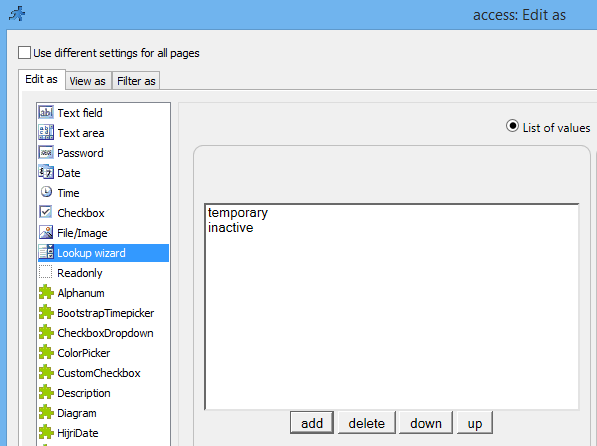Let’s say you need to provide users temporary access to your application. You create a user, set their access to ‘temporary’ and after 24 hours they should not be able to log on to your application. And, of course, you need this to happen automatically.

access – varchar(50). This field will store values like “temporary” or “inactive”. It can be left empty for existing users.

temporary_access_starts – datetime. Indicates when the temporary access starts.

2. Add access field to Add/Edit pages of the login table. Set ‘Edit as’ type to ‘Lookup wizard’ and add two hardcoded values there: “temporary” and “inactive”. Now the admin can create users with temporary access and make them inactive manually.Here we simply initialize temporary_access_starts with the value of the current date/time.

PHP code:

```if(\$values["access"] == "temporary"){
\$values["temporary_access_starts"] = date("Y-m-d H:i:s"); ;
}
return true;```

C# code:

```if(values["access"] == "temporary")
{
values["temporary_access_starts"] = CommonFunctions.date(new XVar("Y-m-d H:i:s"));
}
return true;```

In this event we first check the value of user’s access field. If it says “inactive”, we reject the login. If it says “temporary” and the value of temporary_access_starts field is more than 24 hours old, then we also reject the login. All other users can log on.

PHP code:

```\$user = DB::Select("users", array("username" => \$username))->fetchAssoc();
if (\$user && \$user["access"] == "inactive") {
return false;
}
if (\$user  && \$user["access"] == "temporary") {
\$date_cur = date_create_from_format('"Y-m-d H:i:s"', date('"Y-m-d H:i:s"'));
\$date_end_access = date_create_from_format('"Y-m-d H:i:s"', date('"Y-m-d H:i:s"', strtotime(\$user["temporary_access_starts"])));
\$diff = date_diff(\$date_cur, \$date_end_access);
// >24 hours
if (\$diff->h > 24) return false;
}
return true;```

C# code:

```dynamic user = XVar.Array();
if((XVar)(user)  && (XVar)(user["access"] == "inactive"))
{
return false;
}
if((XVar)(user)  && (XVar)(user["access"] == "temporary"))
{
DateTime startTime = DateTime.Now;
DateTime endTime = Convert.ToDateTime(user["temporary_access_starts"].ToString());
TimeSpan span = endTime.Subtract ( startTime );
if(span.Hours > 24) {
return false;
}
}
return true;```